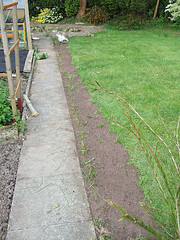# How Many Cubic Feet For A 40lb Bag Of Topsoil?A 40 pound bag will be about one cubic foot. Therefore, a bag measuring one foot in length multiplied by one foot in width multiplied by one foot in height is able to hold forty pounds of topsoil.

One foot is equivalent to twelve inches which, in turn, is equivalent to about 30 centimetres. Therefore one cubic foot is equivalent to 12 inches in length multiplied by 12 inches in width multiplied by 12 inches in height. This multiplication, therefore, is 12 inches in length multiplied by 12 inches in width multiplied by 12 inches in height, which gives us the product of 1, 728 cubic inches.

The measurement of the bag can also be expressed in terms of cubic centimetres. Thirty centimetres in length multiplied by 30 centimetres in width multiplied by 30 centimetres in height is equivalent to 27, 000 cubic centimetres. Therefore, a bag measuring either one cubic foot or 1, 728 cubic inches or 27, 000 cubic centimetres can hold 40 pounds of topsoil.
thanked the writer.
Actually it's closer to .75cf - So, for a bed of 30x20 x 8in deep, you'd need approximately 2.77cf of topsoil which is roughly 3.5 40lbs bags. Depending on where you buy, it can run around \$1.50 a 40lbs bag (\$1.48 @ Home Depot in State College PA as of April 10th)
thanked the writer.
How many bags of topsoil weighing 40lbs will it take to fill an area 30 x 20 feet x 8 ins deep
thanked the writer.